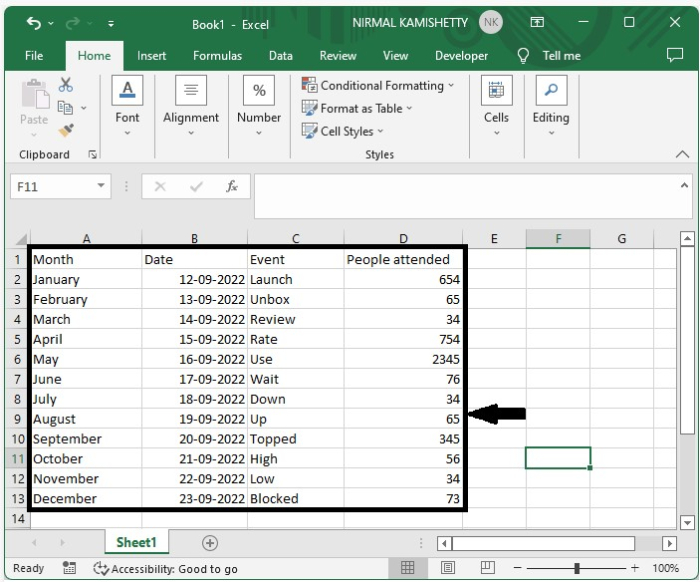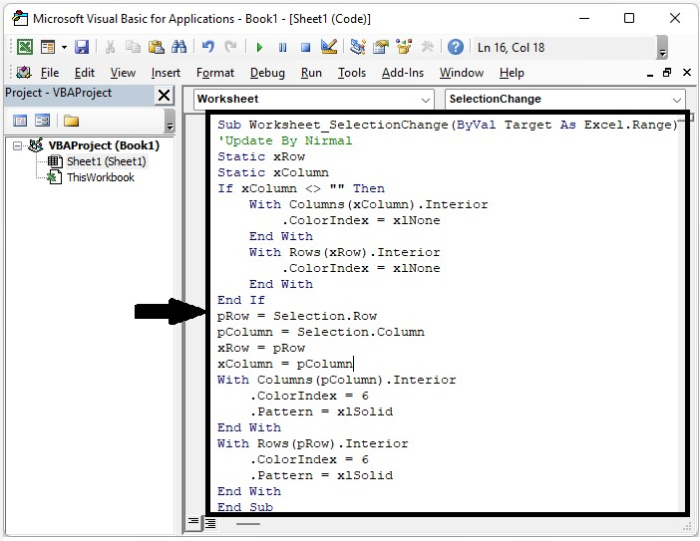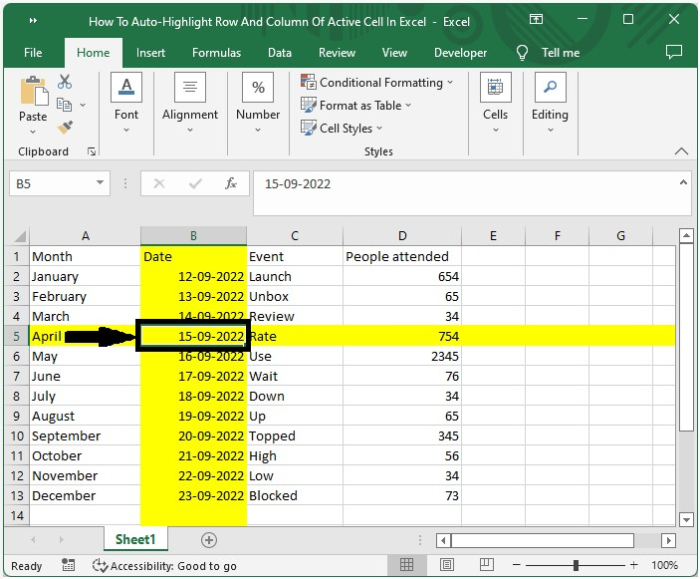# How to Auto-Highlight the Row and Column of an Active Cell in Excel?

When we have a large amount of data on a single visible sheet, we can have the problem of straining our eyes to see the selected value clearly, which leads to a value misreading. But we can solve this problem by highlighting the row and column of active cells with different colours. This tutorial will help you understand how we can automatically highlight rows and columns of active cells in Excel.

## Auto-Highlight Row and Column of an Active Cell

Here we will insert VBA code for the sheet, and then every time we select a cell, the row and column will be highlighted. Let us see a simple process to understand how we can auto-highlight rows and columns if there is an active cell in Excel using the vba application.

Step 1

Consider the following scenario − we have an excel sheet with data that is similar to the data shown in the image below.Now, right-click on the sheet name and select "View Code" to open the VBA application, and type the programme into the sheet as shown in the below image.

### Example

Sub Worksheet_SelectionChange(ByVal Target As Excel.Range)
'Update By Nirmal
Static xRow
Static xColumn
If xColumn <> "" Then
With Columns(xColumn).Interior
.ColorIndex = xlNone
End With
With Rows(xRow).Interior
.ColorIndex = xlNone
End With
End If
pRow = Selection.Row
pColumn = Selection.Column
xRow = pRow
xColumn = pColumn
With Columns(pColumn).Interior
.ColorIndex = 6
.Pattern = xlSolid
End With
With Rows(pRow).Interior
.ColorIndex = 6
.Pattern = xlSolid
End With
End Sub


In the code, the colour code is represented by Colorindex = 6.Step 2

Now save the sheet as a macro-enabled sheet and close the VBA application by pressing "Alt + Q". Every time we click on a cell in Excel, the row and column of the cell will be highlighted, as shown in the below image.## Conclusion

In this tutorial, we used a simple example to demonstrate how we can auto-highlight rows and columns if an active cell in Excel is selected.

Updated on: 10-Jan-2023

447 Views